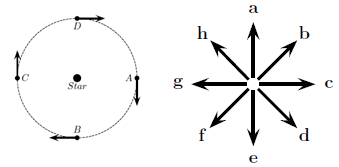# Problem: A planet with a mass of 1 × 1023 kg travels around a star in a nearly circular obrbit in the xy plane as shown in the diagram. Its speed is constant at 15000 m/s. Which arrow best describes the direction of ΔP in going from B to C? 1. g . 2. f 3. c 4. e 5. b 6. d 7. h 8. a

###### FREE Expert Solution
96% (186 ratings)
###### Problem Details

A planet with a mass of 1 × 1023 kg travels around a star in a nearly circular obrbit in the xy plane as shown in the diagram. Its speed is constant at 15000 m/s. Which arrow best describes the direction of ΔP in going from B to C?

1. g .

2. f

3. c

4. e

5. b

6. d

7. h

8. a